Anúncio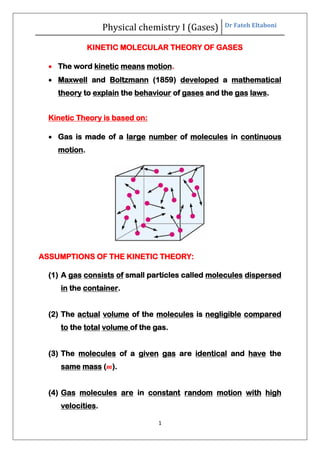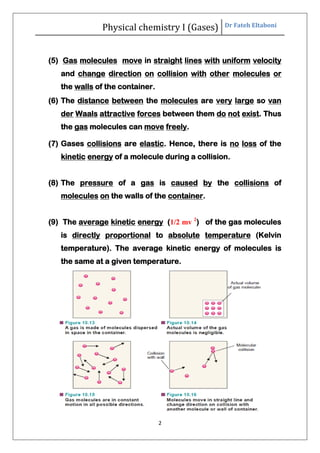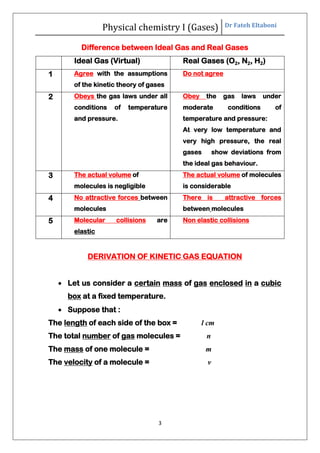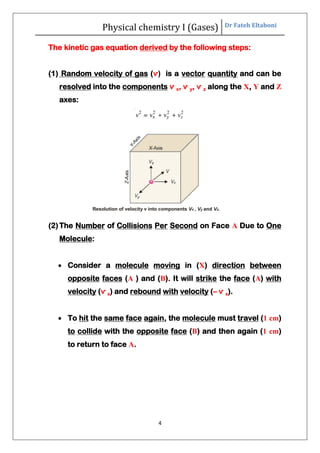Anúncio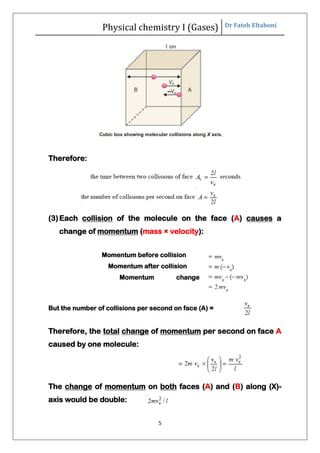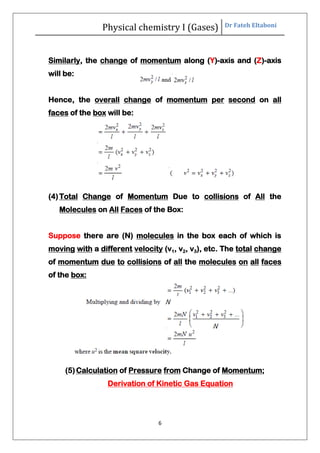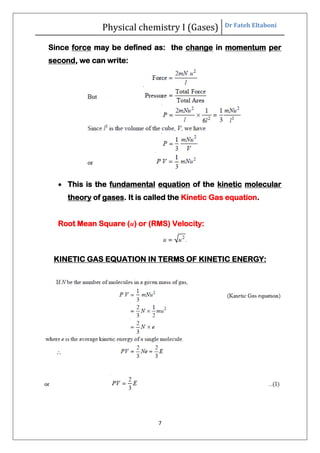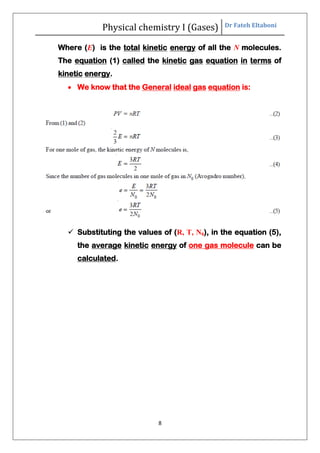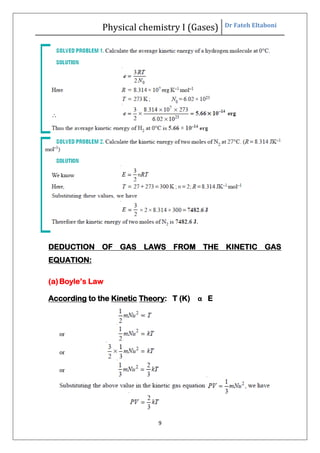Anúncio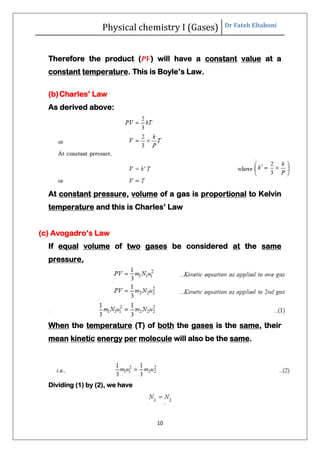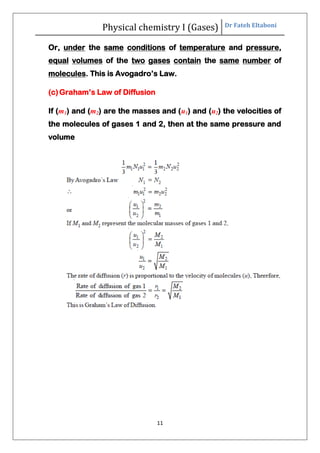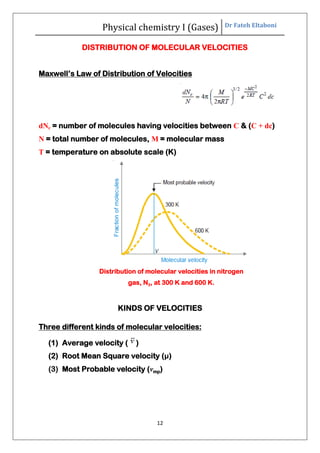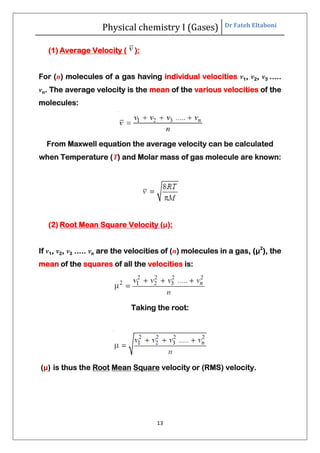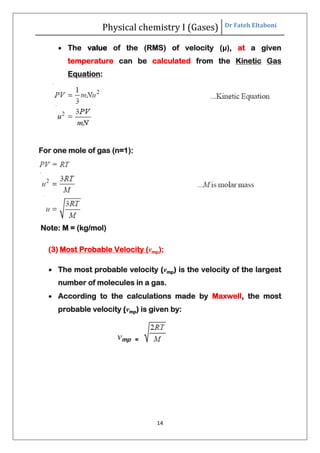Anúncio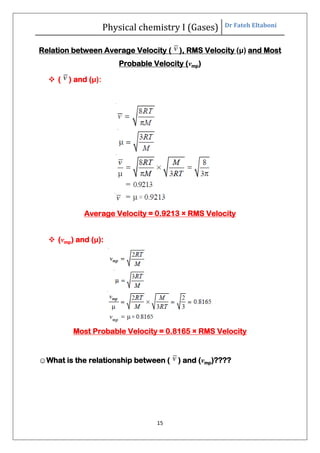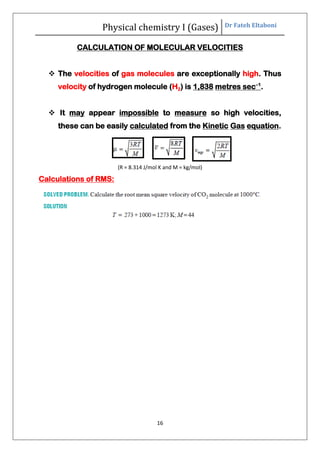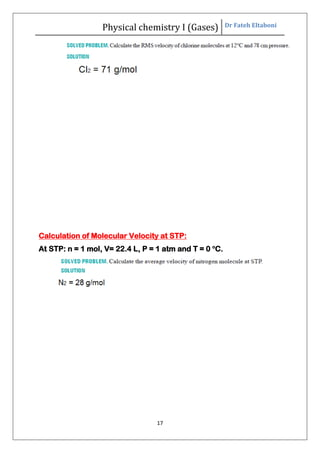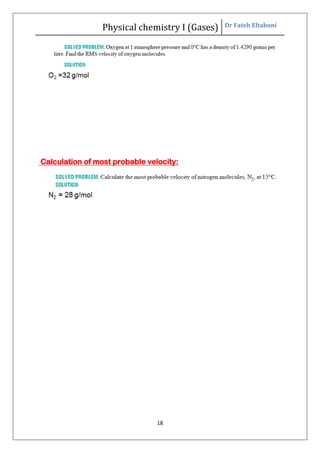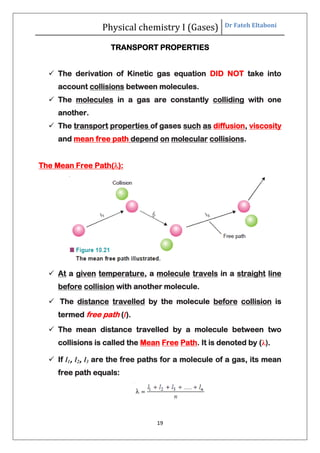Anúncio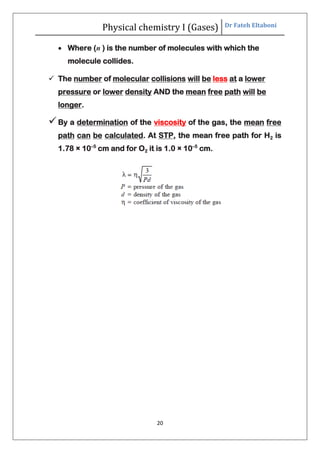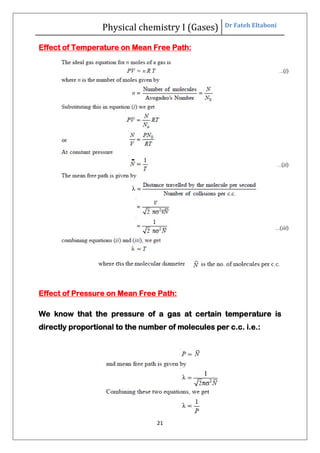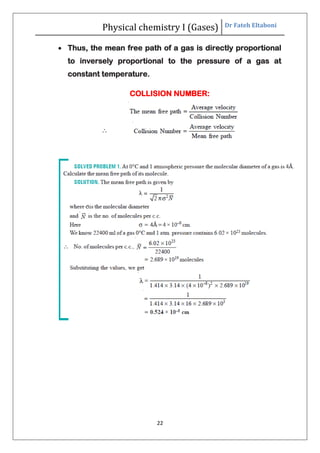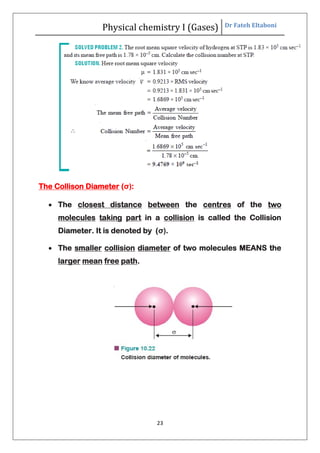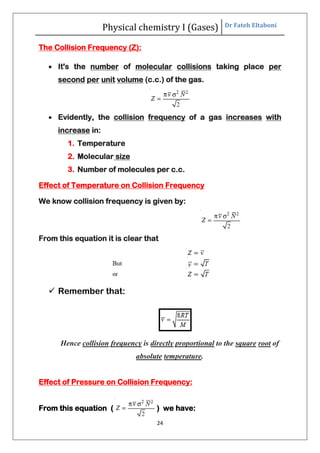Anúncio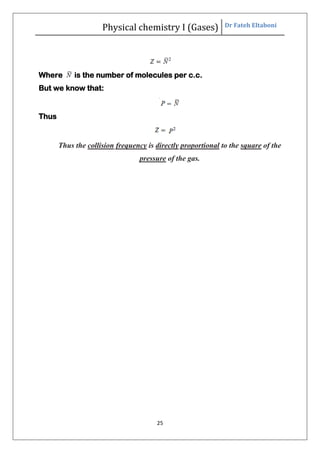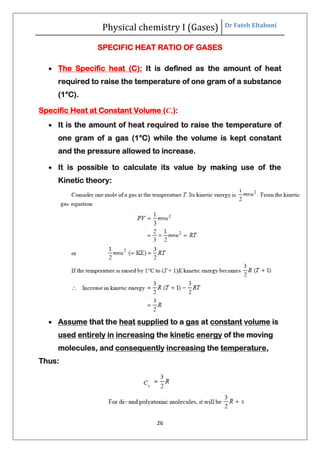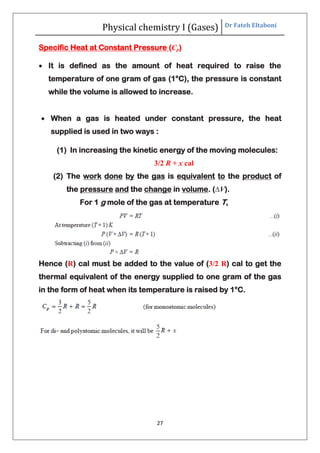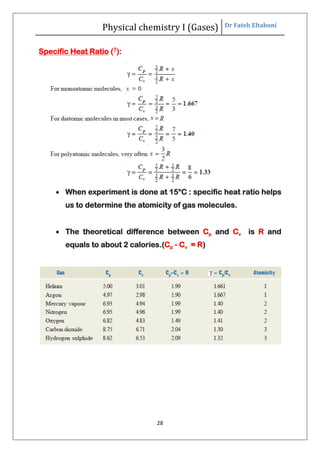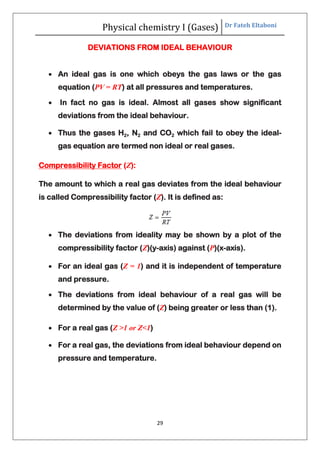Anúncio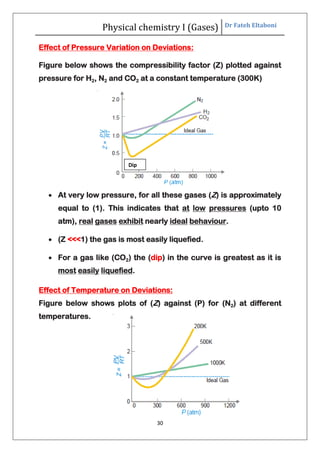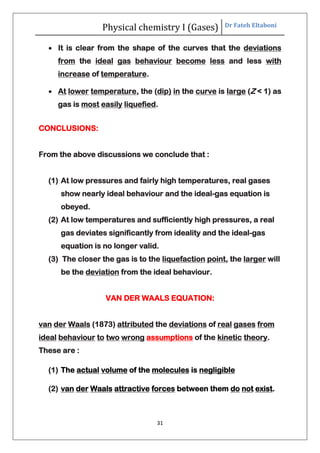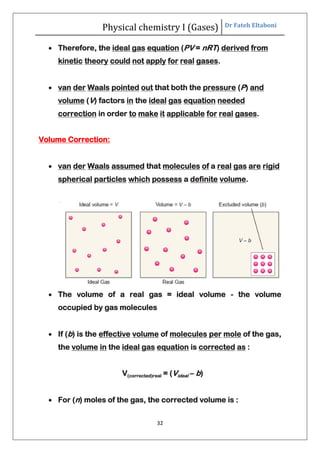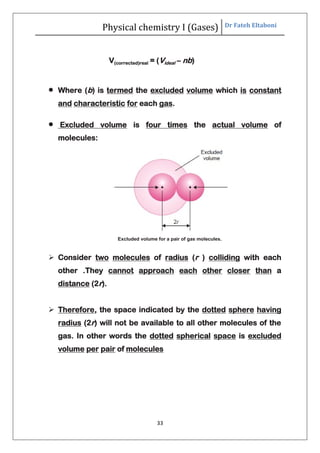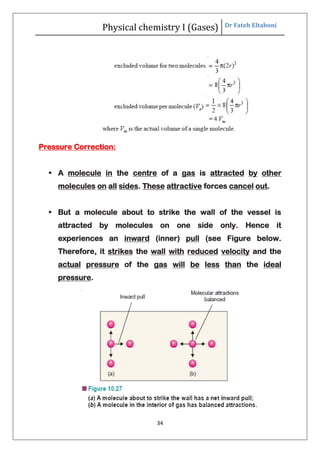Anúncio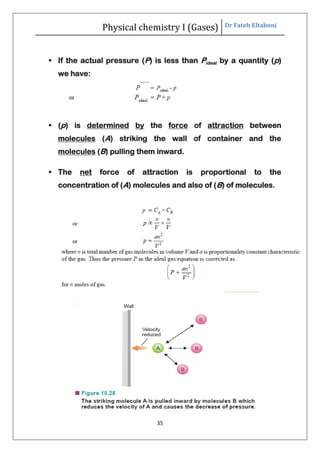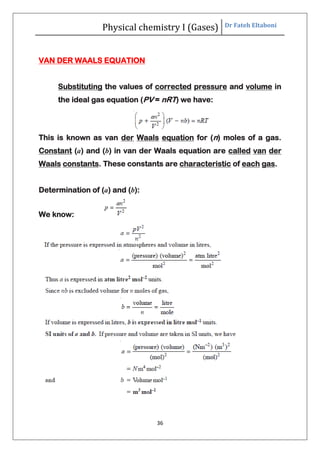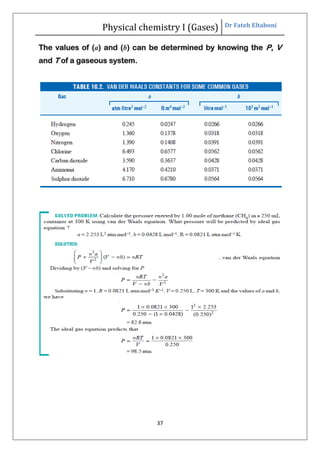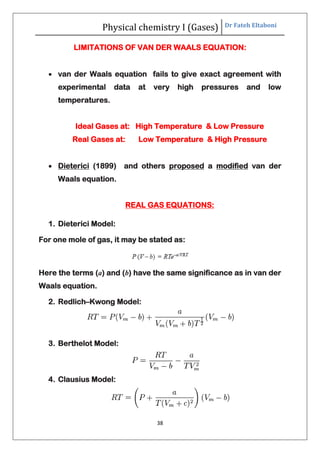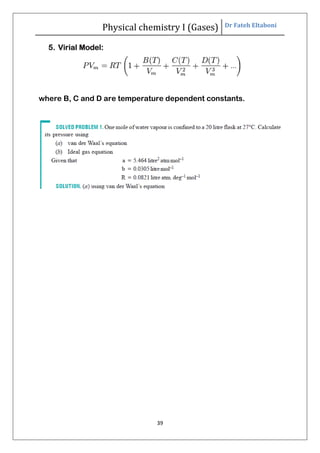Anúncio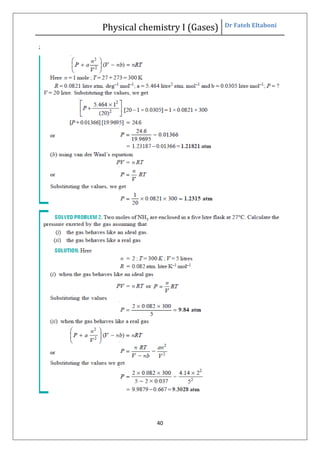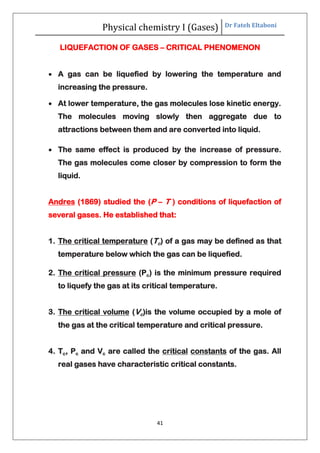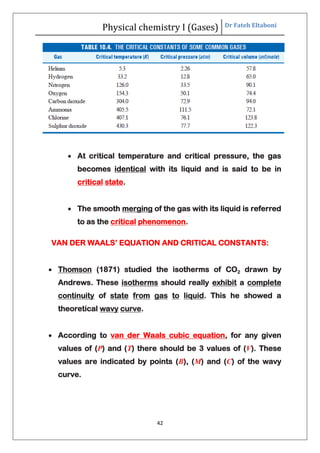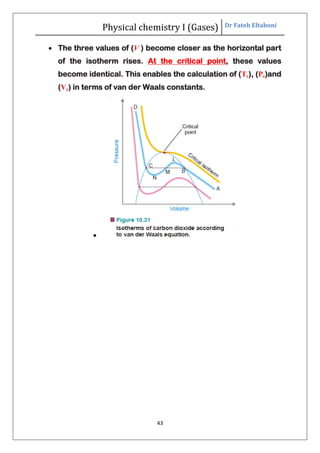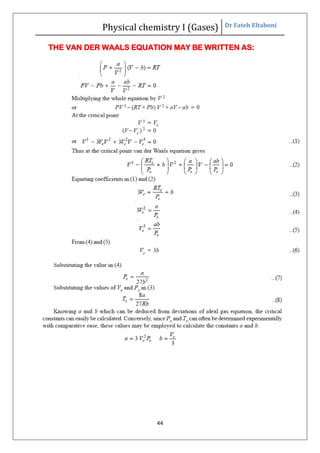Anúncio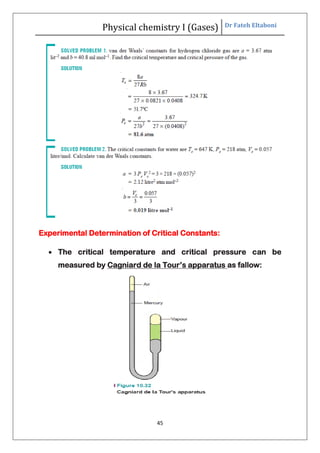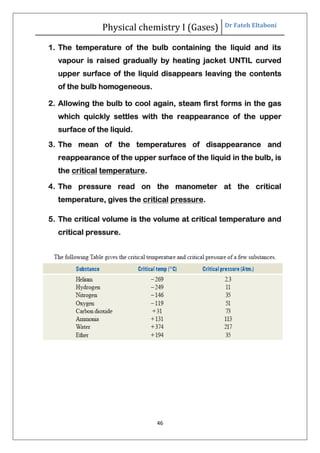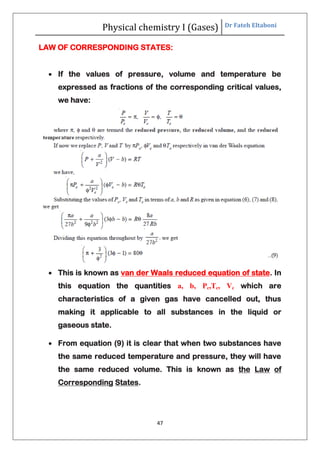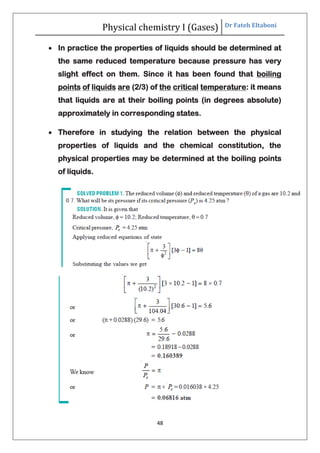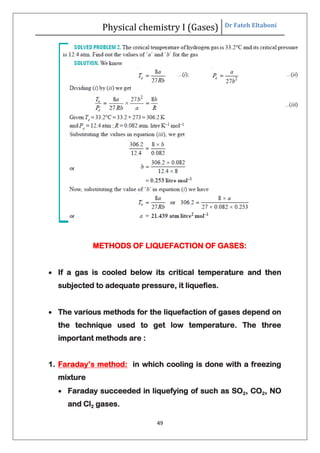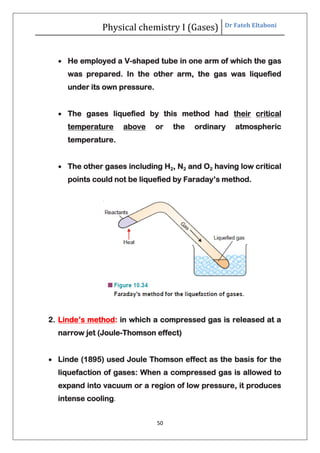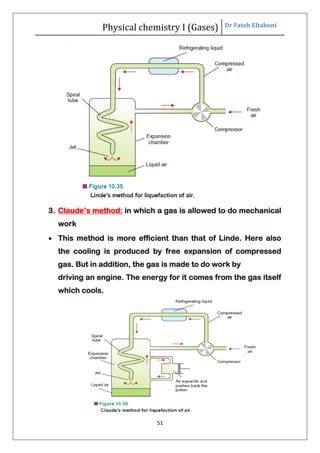Próximos SlideShares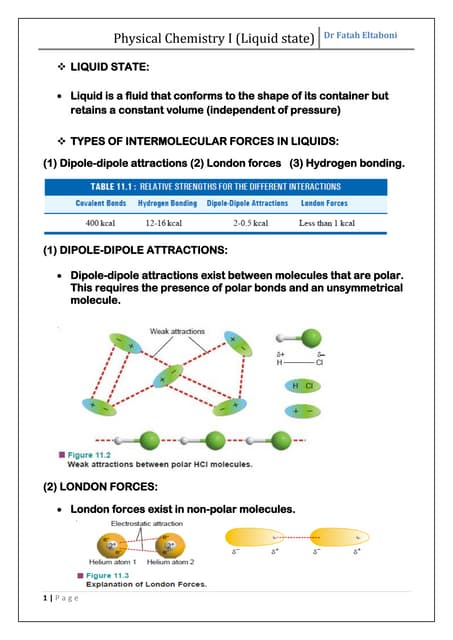Liquid state
Carregando em ... 3
1 de 51
Anúncio

### Gases

1. Physical chemistry I (Gases) Dr Fateh Eltaboni 1 KINETIC MOLECULAR THEORY OF GASES  The word kinetic means motion.  Maxwell and Boltzmann (1859) developed a mathematical theory to explain the behaviour of gases and the gas laws. Kinetic Theory is based on:  Gas is made of a large number of molecules in continuous motion. ASSUMPTIONS OF THE KINETIC THEORY: (1) A gas consists of small particles called molecules dispersed in the container. (2) The actual volume of the molecules is negligible compared to the total volume of the gas. (3) The molecules of a given gas are identical and have the same mass (m). (4) Gas molecules are in constant random motion with high velocities.
2. Physical chemistry I (Gases) Dr Fateh Eltaboni 2 (5) Gas molecules move in straight lines with uniform velocity and change direction on collision with other molecules or the walls of the container. (6) The distance between the molecules are very large so van der Waals attractive forces between them do not exist. Thus the gas molecules can move freely. (7) Gases collisions are elastic. Hence, there is no loss of the kinetic energy of a molecule during a collision. (8) The pressure of a gas is caused by the collisions of molecules on the walls of the container. (9) The average kinetic energy (1/2 mv 2 ) of the gas molecules is directly proportional to absolute temperature (Kelvin temperature). The average kinetic energy of molecules is the same at a given temperature.
3. Physical chemistry I (Gases) Dr Fateh Eltaboni 3 Difference between Ideal Gas and Real Gases Ideal Gas (Virtual) Real Gases (O2, N2, H2) 1 Agree with the assumptions of the kinetic theory of gases Do not agree 2 Obeys the gas laws under all conditions of temperature and pressure. Obey the gas laws under moderate conditions of temperature and pressure: At very low temperature and very high pressure, the real gases show deviations from the ideal gas behaviour. 3 The actual volume of molecules is negligible The actual volume of molecules is considerable 4 No attractive forces between molecules There is attractive forces between molecules 5 Molecular collisions are elastic Non elastic collisions DERIVATION OF KINETIC GAS EQUATION  Let us consider a certain mass of gas enclosed in a cubic box at a fixed temperature.  Suppose that : The length of each side of the box = l cm The total number of gas molecules = n The mass of one molecule = m The velocity of a molecule = v
4. Physical chemistry I (Gases) Dr Fateh Eltaboni 4 The kinetic gas equation derived by the following steps: (1) Random velocity of gas (ѵ) is a vector quantity and can be resolved into the components ѵ x, ѵ y, ѵ z along the X, Y and Z axes: Resolution of velocity v into components Vx , Vy and Vz. (2)The Number of Collisions Per Second on Face A Due to One Molecule:  Consider a molecule moving in (X) direction between opposite faces (A ) and (B). It will strike the face (A) with velocity (ѵ x) and rebound with velocity (– ѵ x).  To hit the same face again, the molecule must travel (1 cm) to collide with the opposite face (B) and then again (1 cm) to return to face A.
5. Physical chemistry I (Gases) Dr Fateh Eltaboni 5 Cubic box showing molecular collisions along X axis. Therefore: (3)Each collision of the molecule on the face (A) causes a change of momentum (mass × velocity): Momentum before collision Momentum after collision Momentum change But the number of collisions per second on face (A) = Therefore, the total change of momentum per second on face A caused by one molecule: The change of momentum on both faces (A) and (B) along (X)- axis would be double:
6. Physical chemistry I (Gases) Dr Fateh Eltaboni 6 Similarly, the change of momentum along (Y)-axis and (Z)-axis will be: Hence, the overall change of momentum per second on all faces of the box will be: (4)Total Change of Momentum Due to collisions of All the Molecules on All Faces of the Box: Suppose there are (N) molecules in the box each of which is moving with a different velocity (v1, v2, v3), etc. The total change of momentum due to collisions of all the molecules on all faces of the box: (5)Calculation of Pressure from Change of Momentum; Derivation of Kinetic Gas Equation
7. Physical chemistry I (Gases) Dr Fateh Eltaboni 7 Since force may be defined as: the change in momentum per second, we can write:  This is the fundamental equation of the kinetic molecular theory of gases. It is called the Kinetic Gas equation. Root Mean Square (u) or (RMS) Velocity: KINETIC GAS EQUATION IN TERMS OF KINETIC ENERGY:
8. Physical chemistry I (Gases) Dr Fateh Eltaboni 8 Where (E) is the total kinetic energy of all the N molecules. The equation (1) called the kinetic gas equation in terms of kinetic energy.  We know that the General ideal gas equation is:  Substituting the values of (R, T, N0), in the equation (5), the average kinetic energy of one gas molecule can be calculated.
9. Physical chemistry I (Gases) Dr Fateh Eltaboni 9 DEDUCTION OF GAS LAWS FROM THE KINETIC GAS EQUATION: (a)Boyle’s Law According to the Kinetic Theory: T (K) α E
10. Physical chemistry I (Gases) Dr Fateh Eltaboni 10 Therefore the product (PV) will have a constant value at a constant temperature. This is Boyle’s Law. (b)Charles’ Law As derived above: At constant pressure, volume of a gas is proportional to Kelvin temperature and this is Charles’ Law (c) Avogadro’s Law If equal volume of two gases be considered at the same pressure, When the temperature (T) of both the gases is the same, their mean kinetic energy per molecule will also be the same. Dividing (1) by (2), we have
11. Physical chemistry I (Gases) Dr Fateh Eltaboni 11 Or, under the same conditions of temperature and pressure, equal volumes of the two gases contain the same number of molecules. This is Avogadro’s Law. (c)Graham’s Law of Diffusion If (m1) and (m2) are the masses and (u1) and (u2) the velocities of the molecules of gases 1 and 2, then at the same pressure and volume
12. Physical chemistry I (Gases) Dr Fateh Eltaboni 12 DISTRIBUTION OF MOLECULAR VELOCITIES Maxwell’s Law of Distribution of Velocities dNc = number of molecules having velocities between C & (C + dc) N = total number of molecules, M = molecular mass T = temperature on absolute scale (K) Distribution of molecular velocities in nitrogen gas, N2, at 300 K and 600 K. KINDS OF VELOCITIES Three different kinds of molecular velocities: (1) Average velocity ( ) (2) Root Mean Square velocity (μ) (3) Most Probable velocity (vmp)
13. Physical chemistry I (Gases) Dr Fateh Eltaboni 13 (1) Average Velocity ( ): For (n) molecules of a gas having individual velocities v1, v2, v3 ..... vn. The average velocity is the mean of the various velocities of the molecules: From Maxwell equation the average velocity can be calculated when Temperature (T) and Molar mass of gas molecule are known: (2) Root Mean Square Velocity (μ): If v1, v2, v3 ..... vn are the velocities of (n) molecules in a gas, (μ2 ), the mean of the squares of all the velocities is: Taking the root: (μ) is thus the Root Mean Square velocity or (RMS) velocity.
14. Physical chemistry I (Gases) Dr Fateh Eltaboni 14  The value of the (RMS) of velocity (μ), at a given temperature can be calculated from the Kinetic Gas Equation: For one mole of gas (n=1): Note: M = (kg/mol) (3) Most Probable Velocity (vmp):  The most probable velocity (vmp) is the velocity of the largest number of molecules in a gas.  According to the calculations made by Maxwell, the most probable velocity (vmp) is given by: vmp =
15. Physical chemistry I (Gases) Dr Fateh Eltaboni 15 Relation between Average Velocity ( ), RMS Velocity (μ) and Most Probable Velocity (vmp)  ( ) and (μ): Average Velocity = 0.9213 × RMS Velocity  (vmp) and (μ): Most Probable Velocity = 0.8165 × RMS Velocity ☺What is the relationship between ( ) and (vmp)????
16. Physical chemistry I (Gases) Dr Fateh Eltaboni 16 CALCULATION OF MOLECULAR VELOCITIES  The velocities of gas molecules are exceptionally high. Thus velocity of hydrogen molecule (H2) is 1,838 metres sec–1 .  It may appear impossible to measure so high velocities, these can be easily calculated from the Kinetic Gas equation. (R = 8.314 J/mol K and M = kg/mol) Calculations of RMS:
17. Physical chemistry I (Gases) Dr Fateh Eltaboni 17 Calculation of Molecular Velocity at STP: At STP: n = 1 mol, V= 22.4 L, P = 1 atm and T = 0 o C.
18. Physical chemistry I (Gases) Dr Fateh Eltaboni 18 Calculation of most probable velocity:
19. Physical chemistry I (Gases) Dr Fateh Eltaboni 19 TRANSPORT PROPERTIES  The derivation of Kinetic gas equation DID NOT take into account collisions between molecules.  The molecules in a gas are constantly colliding with one another.  The transport properties of gases such as diffusion, viscosity and mean free path depend on molecular collisions. The Mean Free Path():  At a given temperature, a molecule travels in a straight line before collision with another molecule.  The distance travelled by the molecule before collision is termed free path (l).  The mean distance travelled by a molecule between two collisions is called the Mean Free Path. It is denoted by (λ).  If l1, l2, l3 are the free paths for a molecule of a gas, its mean free path equals:
20. Physical chemistry I (Gases) Dr Fateh Eltaboni 20  Where (n ) is the number of molecules with which the molecule collides.  The number of molecular collisions will be less at a lower pressure or lower density AND the mean free path will be longer. By a determination of the viscosity of the gas, the mean free path can be calculated. At STP, the mean free path for H2 is 1.78 × 10–5 cm and for O2 it is 1.0 × 10–5 cm.
21. Physical chemistry I (Gases) Dr Fateh Eltaboni 21 Effect of Temperature on Mean Free Path: Effect of Pressure on Mean Free Path: We know that the pressure of a gas at certain temperature is directly proportional to the number of molecules per c.c. i.e.:
22. Physical chemistry I (Gases) Dr Fateh Eltaboni 22  Thus, the mean free path of a gas is directly proportional to inversely proportional to the pressure of a gas at constant temperature. COLLISION NUMBER:
23. Physical chemistry I (Gases) Dr Fateh Eltaboni 23 The Collison Diameter (σ):  The closest distance between the centres of the two molecules taking part in a collision is called the Collision Diameter. It is denoted by (σ).  The smaller collision diameter of two molecules MEANS the larger mean free path.
24. Physical chemistry I (Gases) Dr Fateh Eltaboni 24 The Collision Frequency (Z):  It's the number of molecular collisions taking place per second per unit volume (c.c.) of the gas.  Evidently, the collision frequency of a gas increases with increase in: 1. Temperature 2. Molecular size 3. Number of molecules per c.c. Effect of Temperature on Collision Frequency We know collision frequency is given by: From this equation it is clear that  Remember that: Hence collision frequency is directly proportional to the square root of absolute temperature. Effect of Pressure on Collision Frequency: From this equation ( ) we have:
25. Physical chemistry I (Gases) Dr Fateh Eltaboni 25 Where is the number of molecules per c.c. But we know that: Thus Thus the collision frequency is directly proportional to the square of the pressure of the gas.
26. Physical chemistry I (Gases) Dr Fateh Eltaboni 26 SPECIFIC HEAT RATIO OF GASES  The Specific heat (C): It is defined as the amount of heat required to raise the temperature of one gram of a substance (1°C). Specific Heat at Constant Volume (Cv):  It is the amount of heat required to raise the temperature of one gram of a gas (1°C) while the volume is kept constant and the pressure allowed to increase.  It is possible to calculate its value by making use of the Kinetic theory:  Assume that the heat supplied to a gas at constant volume is used entirely in increasing the kinetic energy of the moving molecules, and consequently increasing the temperature, Thus:
27. Physical chemistry I (Gases) Dr Fateh Eltaboni 27 Specific Heat at Constant Pressure (Cp)  It is defined as the amount of heat required to raise the temperature of one gram of gas (1°C), the pressure is constant while the volume is allowed to increase.  When a gas is heated under constant pressure, the heat supplied is used in two ways : (1) In increasing the kinetic energy of the moving molecules: 3/2 R + x cal (2) The work done by the gas is equivalent to the product of the pressure and the change in volume. (∆V). For 1 g mole of the gas at temperature T, Hence (R) cal must be added to the value of (3/2 R) cal to get the thermal equivalent of the energy supplied to one gram of the gas in the form of heat when its temperature is raised by 1°C.
28. Physical chemistry I (Gases) Dr Fateh Eltaboni 28 Specific Heat Ratio (ᵞ):  When experiment is done at 15°C : specific heat ratio helps us to determine the atomicity of gas molecules.  The theoretical difference between Cp and Cv is R and equals to about 2 calories.(Cp - Cv = R)
29. Physical chemistry I (Gases) Dr Fateh Eltaboni 29 DEVIATIONS FROM IDEAL BEHAVIOUR  An ideal gas is one which obeys the gas laws or the gas equation (PV = RT) at all pressures and temperatures.  In fact no gas is ideal. Almost all gases show significant deviations from the ideal behaviour.  Thus the gases H2, N2 and CO2 which fail to obey the ideal- gas equation are termed non ideal or real gases. Compressibility Factor (Z): The amount to which a real gas deviates from the ideal behaviour is called Compressibility factor (Z). It is defined as:  The deviations from ideality may be shown by a plot of the compressibility factor (Z)(y-axis) against (P)(x-axis).  For an ideal gas (Z = 1) and it is independent of temperature and pressure.  The deviations from ideal behaviour of a real gas will be determined by the value of (Z) being greater or less than (1).  For a real gas (Z >1 or Z<1)  For a real gas, the deviations from ideal behaviour depend on pressure and temperature.
30. Physical chemistry I (Gases) Dr Fateh Eltaboni 30 Effect of Pressure Variation on Deviations: Figure below shows the compressibility factor (Z) plotted against pressure for H2, N2 and CO2 at a constant temperature (300K)  At very low pressure, for all these gases (Z) is approximately equal to (1). This indicates that at low pressures (upto 10 atm), real gases exhibit nearly ideal behaviour.  (Z <<<1) the gas is most easily liquefied.  For a gas like (CO2) the (dip) in the curve is greatest as it is most easily liquefied. Effect of Temperature on Deviations: Figure below shows plots of (Z) against (P) for (N2) at different temperatures. Dip
31. Physical chemistry I (Gases) Dr Fateh Eltaboni 31  It is clear from the shape of the curves that the deviations from the ideal gas behaviour become less and less with increase of temperature.  At lower temperature, the (dip) in the curve is large (Z < 1) as gas is most easily liquefied. CONCLUSIONS: From the above discussions we conclude that : (1) At low pressures and fairly high temperatures, real gases show nearly ideal behaviour and the ideal-gas equation is obeyed. (2) At low temperatures and sufficiently high pressures, a real gas deviates significantly from ideality and the ideal-gas equation is no longer valid. (3) The closer the gas is to the liquefaction point, the larger will be the deviation from the ideal behaviour. VAN DER WAALS EQUATION: van der Waals (1873) attributed the deviations of real gases from ideal behaviour to two wrong assumptions of the kinetic theory. These are : (1) The actual volume of the molecules is negligible (2) van der Waals attractive forces between them do not exist.
32. Physical chemistry I (Gases) Dr Fateh Eltaboni 32  Therefore, the ideal gas equation (PV = nRT) derived from kinetic theory could not apply for real gases.  van der Waals pointed out that both the pressure (P) and volume (V) factors in the ideal gas equation needed correction in order to make it applicable for real gases. Volume Correction:  van der Waals assumed that molecules of a real gas are rigid spherical particles which possess a definite volume.  The volume of a real gas = ideal volume - the volume occupied by gas molecules  If (b) is the effective volume of molecules per mole of the gas, the volume in the ideal gas equation is corrected as : V(corrected)real = (Videal – b)  For (n) moles of the gas, the corrected volume is :
33. Physical chemistry I (Gases) Dr Fateh Eltaboni 33 V(corrected)real = (Videal – nb)  Where (b) is termed the excluded volume which is constant and characteristic for each gas.  Excluded volume is four times the actual volume of molecules: Excluded volume for a pair of gas molecules.  Consider two molecules of radius (r ) colliding with each other .They cannot approach each other closer than a distance (2r).  Therefore, the space indicated by the dotted sphere having radius (2r) will not be available to all other molecules of the gas. In other words the dotted spherical space is excluded volume per pair of molecules
34. Physical chemistry I (Gases) Dr Fateh Eltaboni 34 Pressure Correction:  A molecule in the centre of a gas is attracted by other molecules on all sides. These attractive forces cancel out.  But a molecule about to strike the wall of the vessel is attracted by molecules on one side only. Hence it experiences an inward (inner) pull (see Figure below. Therefore, it strikes the wall with reduced velocity and the actual pressure of the gas will be less than the ideal pressure.
35. Physical chemistry I (Gases) Dr Fateh Eltaboni 35  If the actual pressure (P) is less than Pideal by a quantity (p) we have:  (p) is determined by the force of attraction between molecules (A) striking the wall of container and the molecules (B) pulling them inward.  The net force of attraction is proportional to the concentration of (A) molecules and also of (B) of molecules.
36. Physical chemistry I (Gases) Dr Fateh Eltaboni 36 VAN DER WAALS EQUATION Substituting the values of corrected pressure and volume in the ideal gas equation (PV = nRT) we have: This is known as van der Waals equation for (n) moles of a gas. Constant (a) and (b) in van der Waals equation are called van der Waals constants. These constants are characteristic of each gas. Determination of (a) and (b): We know:
37. Physical chemistry I (Gases) Dr Fateh Eltaboni 37 The values of (a) and (b) can be determined by knowing the P, V and T of a gaseous system.
38. Physical chemistry I (Gases) Dr Fateh Eltaboni 38 LIMITATIONS OF VAN DER WAALS EQUATION:  van der Waals equation fails to give exact agreement with experimental data at very high pressures and low temperatures. Ideal Gases at: High Temperature & Low Pressure Real Gases at: Low Temperature & High Pressure  Dieterici (1899) and others proposed a modified van der Waals equation. REAL GAS EQUATIONS: 1. Dieterici Model: For one mole of gas, it may be stated as: Here the terms (a) and (b) have the same significance as in van der Waals equation. 2. Redlich–Kwong Model: 3. Berthelot Model: 4. Clausius Model:
39. Physical chemistry I (Gases) Dr Fateh Eltaboni 39 5. Virial Model: where B, C and D are temperature dependent constants.
40. Physical chemistry I (Gases) Dr Fateh Eltaboni 40 ;
41. Physical chemistry I (Gases) Dr Fateh Eltaboni 41 LIQUEFACTION OF GASES – CRITICAL PHENOMENON  A gas can be liquefied by lowering the temperature and increasing the pressure.  At lower temperature, the gas molecules lose kinetic energy. The molecules moving slowly then aggregate due to attractions between them and are converted into liquid.  The same effect is produced by the increase of pressure. The gas molecules come closer by compression to form the liquid. Andres (1869) studied the (P – T ) conditions of liquefaction of several gases. He established that: 1. The critical temperature (Tc) of a gas may be defined as that temperature below which the gas can be liquefied. 2. The critical pressure (Pc) is the minimum pressure required to liquefy the gas at its critical temperature. 3. The critical volume (Vc)is the volume occupied by a mole of the gas at the critical temperature and critical pressure. 4. Tc, Pc and Vc are called the critical constants of the gas. All real gases have characteristic critical constants.
42. Physical chemistry I (Gases) Dr Fateh Eltaboni 42  At critical temperature and critical pressure, the gas becomes identical with its liquid and is said to be in critical state.  The smooth merging of the gas with its liquid is referred to as the critical phenomenon. VAN DER WAALS’ EQUATION AND CRITICAL CONSTANTS:  Thomson (1871) studied the isotherms of CO2 drawn by Andrews. These isotherms should really exhibit a complete continuity of state from gas to liquid. This he showed a theoretical wavy curve.  According to van der Waals cubic equation, for any given values of (P) and (T) there should be 3 values of (V). These values are indicated by points (B), (M) and (C) of the wavy curve.
43. Physical chemistry I (Gases) Dr Fateh Eltaboni 43  The three values of (V ) become closer as the horizontal part of the isotherm rises. At the critical point, these values become identical. This enables the calculation of (Tc), (Pc)and (Vc) in terms of van der Waals constants. 
44. Physical chemistry I (Gases) Dr Fateh Eltaboni 44 THE VAN DER WAALS EQUATION MAY BE WRITTEN AS:
45. Physical chemistry I (Gases) Dr Fateh Eltaboni 45 Experimental Determination of Critical Constants:  The critical temperature and critical pressure can be measured by Cagniard de la Tour’s apparatus as fallow:
46. Physical chemistry I (Gases) Dr Fateh Eltaboni 46 1. The temperature of the bulb containing the liquid and its vapour is raised gradually by heating jacket UNTIL curved upper surface of the liquid disappears leaving the contents of the bulb homogeneous. 2. Allowing the bulb to cool again, steam first forms in the gas which quickly settles with the reappearance of the upper surface of the liquid. 3. The mean of the temperatures of disappearance and reappearance of the upper surface of the liquid in the bulb, is the critical temperature. 4. The pressure read on the manometer at the critical temperature, gives the critical pressure. 5. The critical volume is the volume at critical temperature and critical pressure.
47. Physical chemistry I (Gases) Dr Fateh Eltaboni 47 LAW OF CORRESPONDING STATES:  If the values of pressure, volume and temperature be expressed as fractions of the corresponding critical values, we have:  This is known as van der Waals reduced equation of state. In this equation the quantities a, b, Pc,Tc, Vc which are characteristics of a given gas have cancelled out, thus making it applicable to all substances in the liquid or gaseous state.  From equation (9) it is clear that when two substances have the same reduced temperature and pressure, they will have the same reduced volume. This is known as the Law of Corresponding States.
48. Physical chemistry I (Gases) Dr Fateh Eltaboni 48  In practice the properties of liquids should be determined at the same reduced temperature because pressure has very slight effect on them. Since it has been found that boiling points of liquids are (2/3) of the critical temperature: it means that liquids are at their boiling points (in degrees absolute) approximately in corresponding states.  Therefore in studying the relation between the physical properties of liquids and the chemical constitution, the physical properties may be determined at the boiling points of liquids.
49. Physical chemistry I (Gases) Dr Fateh Eltaboni 49 METHODS OF LIQUEFACTION OF GASES:  If a gas is cooled below its critical temperature and then subjected to adequate pressure, it liquefies.  The various methods for the liquefaction of gases depend on the technique used to get low temperature. The three important methods are : 1. Faraday’s method: in which cooling is done with a freezing mixture  Faraday succeeded in liquefying of such as SO2, CO2, NO and Cl2 gases.
50. Physical chemistry I (Gases) Dr Fateh Eltaboni 50  He employed a V-shaped tube in one arm of which the gas was prepared. In the other arm, the gas was liquefied under its own pressure.  The gases liquefied by this method had their critical temperature above or the ordinary atmospheric temperature.  The other gases including H2, N2 and O2 having low critical points could not be liquefied by Faraday’s method. 2. Linde’s method: in which a compressed gas is released at a narrow jet (Joule-Thomson effect)  Linde (1895) used Joule Thomson effect as the basis for the liquefaction of gases: When a compressed gas is allowed to expand into vacuum or a region of low pressure, it produces intense cooling.
51. Physical chemistry I (Gases) Dr Fateh Eltaboni 51 3. Claude’s method: in which a gas is allowed to do mechanical work  This method is more efficient than that of Linde. Here also the cooling is produced by free expansion of compressed gas. But in addition, the gas is made to do work by driving an engine. The energy for it comes from the gas itself which cools.
Anúncio